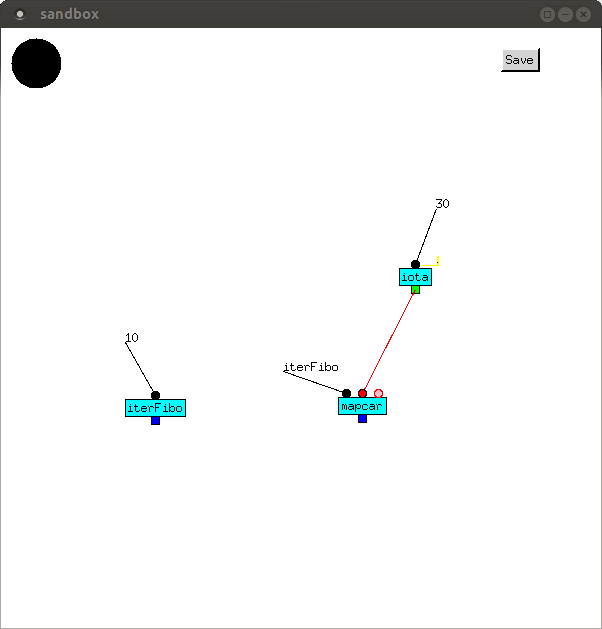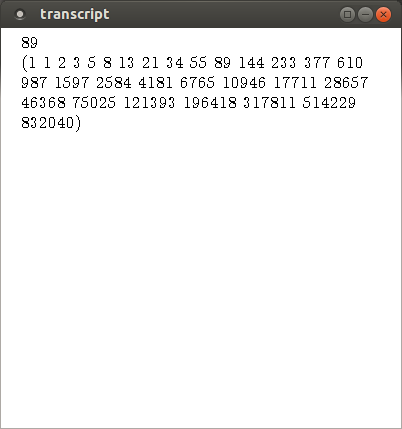# Iteration Again

## Iterative reverse and append

The iterative version of reverse is shown below. It should be compared to `tailRecReverse`. The difference is that, instead of the tail-recursive call, there is a `start+``repeat+` feedback loop, circulating two values. The first is a list which initially arrives on the first input of `iterReverse`, with each iteration removing another element. The value is a list which initially arrives on the second input, with each iteration adding the element to it. When the first value is NIL, the value is returned.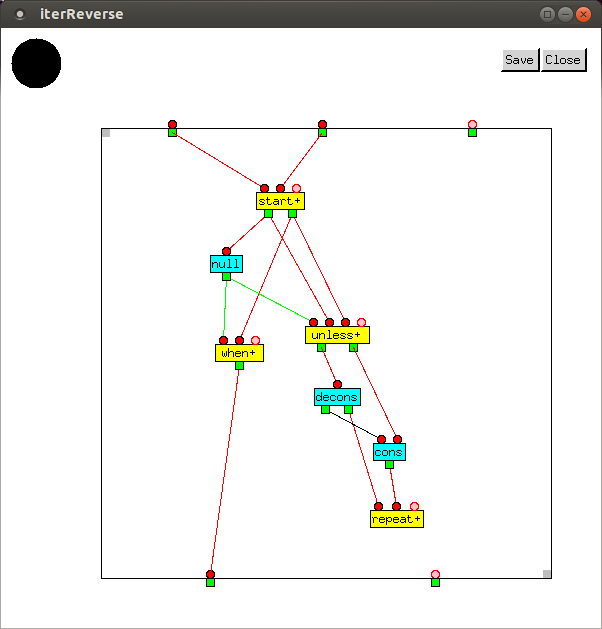The equivalent Lisp function is

```(defun iterReverse (x y)
(do ((p x (cdr p))
(q y (cons (car p) q)))
((null p) q)))
```

`iterReverse` can now be used instead of `tailRecReverse` in `iterAppend`, our new implementation of append.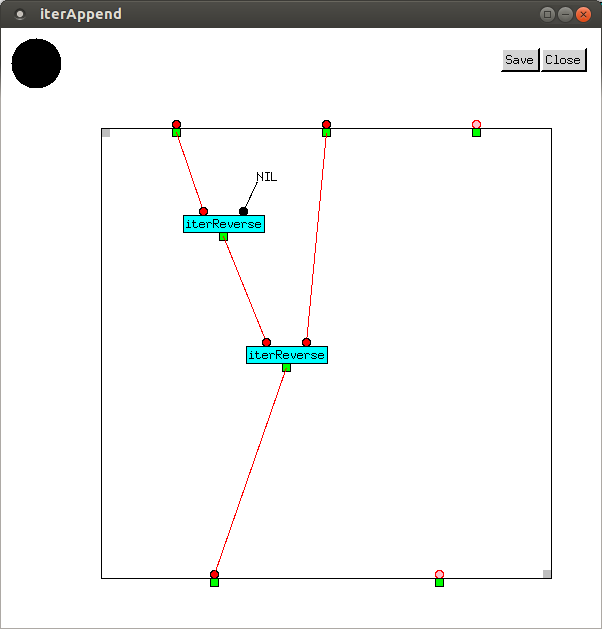The output of the two functions is shown below.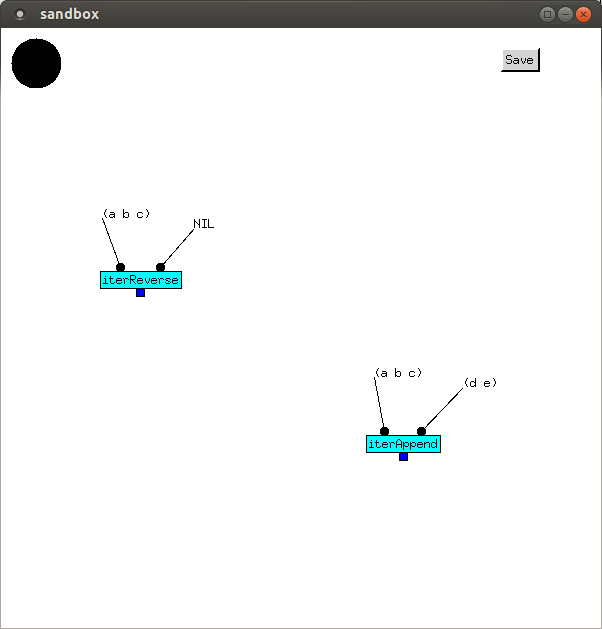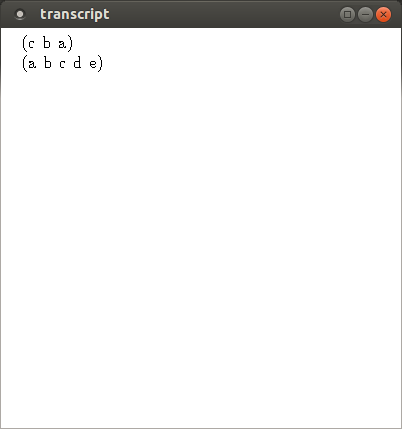## Iterative factorial

The pattern shown in `iterReverse` is repeated in `iterFac`, an iterative implementation of the factorial function. The new thing here is `valve+`, which outputs the values received by its inputs, with the exception of its first value. Its purpose is to provide constant values precisely once to `start+`. Providing them directly to `start+` would result in their being output again whenever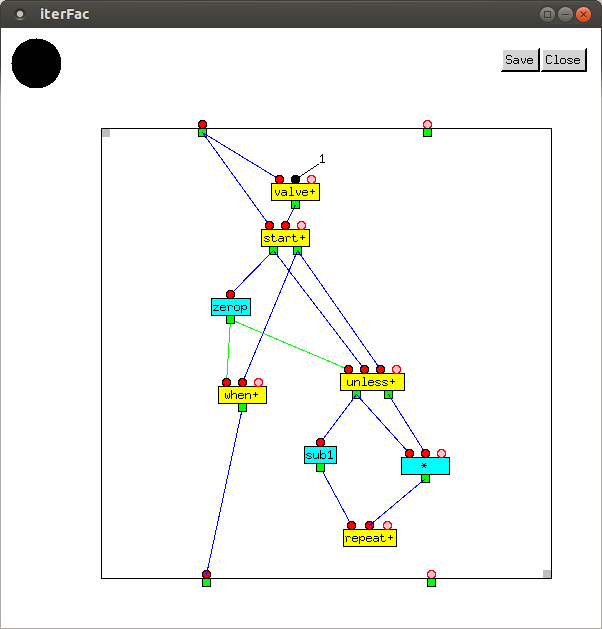The equivalent Lisp function is

```(defun iterFac (n)
(do ((i n (sub1 i))
(f 1 (* i f)))
((zerop i) f)))
```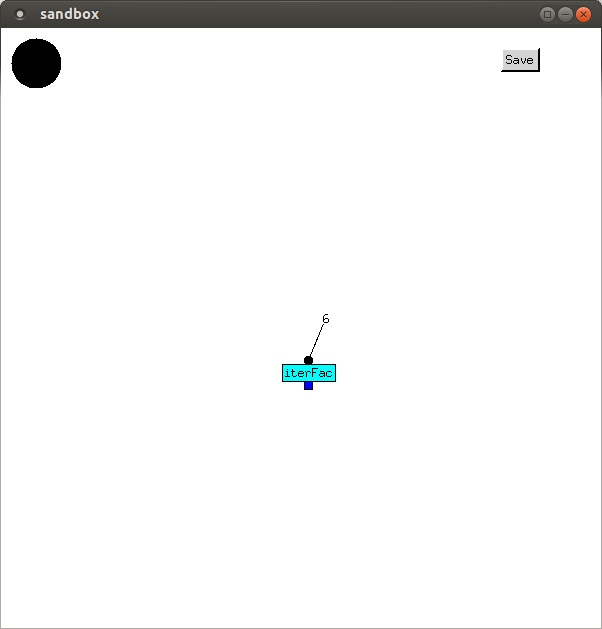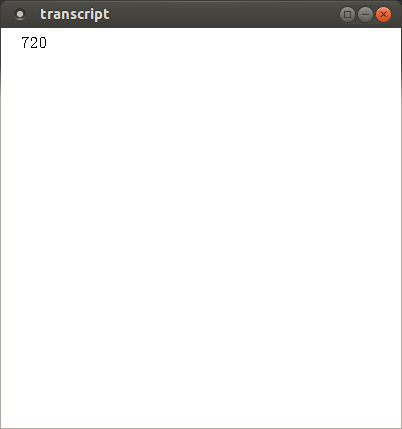## Iterative Fibonacci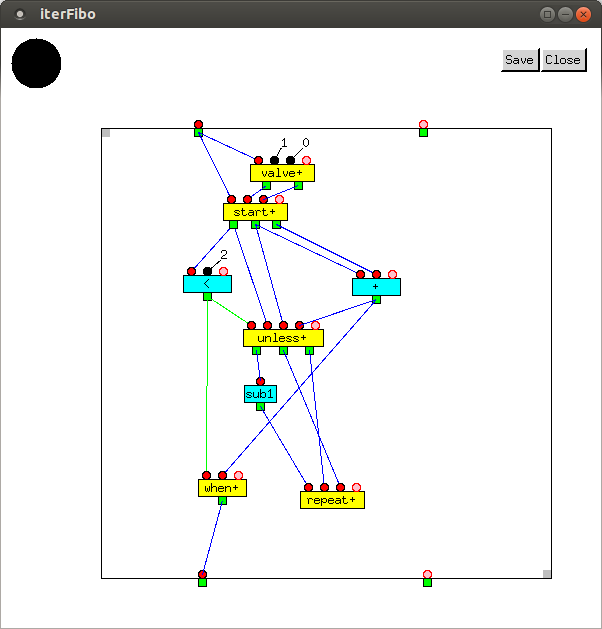The equivalent Lisp function is

```(defun iterFibo (n)
(do ((i n (sub1 i))
(j 1 (+ j k))
(k 0 j))
((< i 2) (+ j k))))
```

`fibo` is called with input 10, followed by a call to `fibo` mapped over the output of `iota` with input 30, which is a list of integers from 0 up to, but not including, 30.